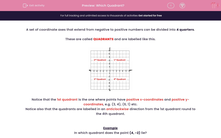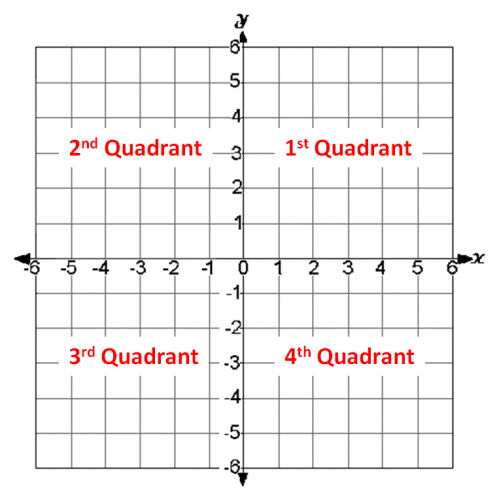### Comprehensive & curriculum aligned

In this worksheet, students must say in which quadrant the given coordinates lie.This content is premium and exclusive to EdPlace subscribers.Key stage:  KS 2

Curriculum topic:   Maths and Numerical Reasoning

Curriculum subtopic:   Equations and Algebra

Difficulty level:#### Worksheet Overview

A set of coordinate axes that extend from negative to positive numbers can be divided into 4 quarters.

These are called QUADRANTS and are labelled like this.Notice that the 1st quadrant is the one where points have positive x-coordinates and positive y-coordinates, e.g. (3, 4), (6, 1) etc.

Notice also that the quadrants are labelled in an anticlockwise direction from the 1st quadrant round to the 4th quadrant.

Example

In which quadrant does the point (4, -2) lie?

(4, -2) has a positive x-coordinate and and a negative y-coordinate.It is in the 4th quadrant.

### What is EdPlace?

We're your National Curriculum aligned online education content provider helping each child succeed in English, maths and science from year 1 to GCSE. With an EdPlace account you’ll be able to track and measure progress, helping each child achieve their best. We build confidence and attainment by personalising each child’s learning at a level that suits them.

Get started